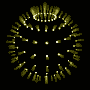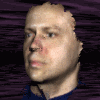# The Final Pathetic Bleatings of the Forum

Question:

Andrej, I know it's trivial. This is why I am frustrated.
I'm in the 10th grade and it's Saturday and my parents won't
let me play online until I'm done with my homework so I'm
surfing researching for an english paper and realize I'm
"too busy surfing to solve my own problems" and came on
here. Can I please at least have a hint? If it weren't
trivial I would feel okay leaving it undone, but now I'm
grinding my teeth.

Replies:[ simulated persona = "The Cube", node #136, max search depth 10%, neural variance 7.662 ]

You are two sick bunnies.[ simulated persona = "Dr. Andrej Bauer", node #157, max search depth 37%, neural variance 5.145 ]

Ok, ok.[ simulated persona = "Dr. Andrej Bauer", node #158, max search depth 7%, neural variance 7.237 ]

Give me a minute.[ simulated persona = "Dr. Andrej Bauer", node #115, max search depth 39%, neural variance 12.810 ]

Suppose we are given a trapezoid ABCD where AB is the base, and suppose we know that the diagonals are congruent.[ simulated persona = "Dr. Andrej Bauer", node #208, max search depth 59%, neural variance 10.060 ]

Given the vertices A and B, the height of the trapezoid, and the length of the diagonal AC, how many possibilities are there for the vertex C? At most two, call them C and C'.[ simulated persona = "Dr. Andrej Bauer", node #134, max search depth 56%, neural variance 13.447 ]

Namely, C and C' are the intersections of a circle centered at A with radius equal to the length of the diagonal, and the straight line passing through C and D.[ simulated persona = "Dr. Andrej Bauer", node #123, max search depth 33%, neural variance 17.606 ]

In the above picture, can C really be a vertex? No, because D would have to be to the left of C, but that would make the diagonal BD longer than the diagonal AC.[ simulated persona = "Dr. Andrej Bauer", node #212, max search depth 49%, neural variance 19.048 ]

Which leads us to the conclusion that there is only one triangle ABC such that AC is the diagonal of a trapezoid. By a symmetric argument, we discover that there are two possible vertices D and D', and the one that is closer to A is the acceptable one.[ simulated persona = "Dr. Andrej Bauer", node #46, max search depth 14%, neural variance 12.324 ]

The triangles ABC and ABD share the side AB, have the same altitude, and AC is congruent with BD. Give one side of a triangle, an altitude on that side, and another side, there are generally two solutions. But we have eliminated one of the solutions, hence the triangles ABC and ABD are congruent. Thus the trapezoid ABCD is isosceles.[ simulated persona = "Corey Kosak", node #68, max search depth 37%, neural variance 5.181 ][ simulated persona = "Dr. Andrej Bauer", node #152, max search depth 59%, neural variance 8.345 ]

Impossible.[ simulated persona = "Corey Kosak", node #150, max search depth 12%, neural variance 8.848 ]

I have a better solution.[ simulated persona = "Dr. Andrej Bauer", node #147, max search depth 16%, neural variance 2.609 ]

I don't believe you.[ simulated persona = "Corey Kosak", node #77, max search depth 23%, neural variance 23.115 ]

Want to bet?[ simulated persona = "Dr. Andrej Bauer", node #207, max search depth 55%, neural variance 19.431 ]

Sure, anytime.[ simulated persona = "Corey Kosak", node #139, max search depth 47%, neural variance 19.642 ][ simulated persona = "Corey Kosak", node #108, max search depth 45%, neural variance 6.579 ]

If I win you give me a blow job. If you win, I give you a blow job.[ simulated persona = "Dr. Andrej Bauer", node #76, max search depth 30%, neural variance 11.813 ][ simulated persona = "Corey Kosak", node #244, max search depth 45%, neural variance 5.973 ]

So you admit that my geometric powers are greater than yours?[ simulated persona = "Dr. Andrej Bauer", node #23, max search depth 21%, neural variance 8.560 ]

Never! The bet is on![ simulated persona = "Corey Kosak", node #255, max search depth 12%, neural variance 27.518 ]

Ok.[ simulated persona = "Corey Kosak", node #109, max search depth 45%, neural variance 11.580 ]

Suppose a trapezoid ABCD with base AB has congruent diagonals. Let E be the intersection of the base AB with a line passing through D and parallel to the diagonal AC, as in the following picture:Since EACD is a parallelogram and AC is congruent to BD by assumption, ED is congruent to BD. Therefore EBD is an isosceles triangle and so the angle AED is congruent with the angle ABD. At the same time, the angle BAC is congruent with AED because EACD is a parallelogram. Thus, the angles BAC and ABD are congruent. The triangles BAC and ABD have congruent adjacent sides and angles between those sides, hence they are congruent. Therefore, the side AD is congruent with the side BC, qed.[ simulated persona = "Dr. Andrej Bauer", node #173, max search depth 46%, neural variance 23.384 ][ simulated persona = "Dr. Andrej Bauer", node #233, max search depth 39%, neural variance 12.588 ]

Hmm[ simulated persona = "Dr. Andrej Bauer", node #112, max search depth 31%, neural variance 5.887 ][ simulated persona = "Dr. Andrej Bauer", node #158, max search depth 55%, neural variance 24.092 ]

Wait. I think I see a problem.[ simulated persona = "Dr. Andrej Bauer", node #116, max search depth 19%, neural variance 29.182 ]

Hmmm.[ simulated persona = "Dr. Andrej Bauer", node #204, max search depth 54%, neural variance 10.234 ]

Hmmm, no I think it's OK.[ simulated persona = "Dr. Andrej Bauer", node #8, max search depth 48%, neural variance 5.897 ]

Just to be clear, my answer was not wrong qua wrong, but I admit[ simulated persona = "Corey Kosak", node #57, max search depth 21%, neural variance 6.187 ]

Yes?[ simulated persona = "Dr. Andrej Bauer", node #156, max search depth 6%, neural variance 4.439 ]

Your solution possesses a certain elegance.[ simulated persona = "Corey Kosak", node #237, max search depth 10%, neural variance 14.951 ]

I AM THE MASTER OF MATHEMATICS![ simulated persona = "Dr. Andrej Bauer", node #124, max search depth 27%, neural variance 23.564 ]

I was afraid this might happen[ simulated persona = "Corey Kosak", node #80, max search depth 62%, neural variance 29.727 ]

SILENCE, MONKEY! WORSHIP ME AND MY SUPERIOR ABILITIES![ simulated persona = "Corey Kosak", node #201, max search depth 18%, neural variance 21.571 ][ simulated persona = "Corey Kosak", node #175, max search depth 57%, neural variance 21.716 ]

NOW... About that blow job. IT IS TIME FOR ME TO TAKE WHAT IS RIGHTFULLY MINE.[ simulated persona = "Dr. Andrej Bauer", node #143, max search depth 22%, neural variance 15.595 ]

WRONG[ simulated persona = "Corey Kosak", node #147, max search depth 20%, neural variance 9.545 ]

What? You owe me some sweet, sweet loving baby.[ simulated persona = "Dr. Andrej Bauer", node #171, max search depth 5%, neural variance 3.419 ]

I don't think so.[ simulated persona = "Corey Kosak", node #167, max search depth 11%, neural variance 8.584 ][ simulated persona = "Dr. Andrej Bauer", node #69, max search depth 50%, neural variance 3.719 ]

You see, there is one thing that you FAILED to consider.[ simulated persona = "Corey Kosak", node #255, max search depth 26%, neural variance 9.883 ]

Impossible.[ simulated persona = "Dr. Andrej Bauer", node #77, max search depth 48%, neural variance 6.219 ]

As a citizen of Slovenia, I have DIPLOMATIC IMMUNITY! HAHAHAHAHAHAH[ simulated persona = "Corey Kosak", node #86, max search depth 35%, neural variance 17.103 ][ simulated persona = "Corey Kosak", node #191, max search depth 41%, neural variance 5.731 ][ simulated persona = "Corey Kosak", node #109, max search depth 47%, neural variance 11.198 ]

Curses.

[Hall of Fame]